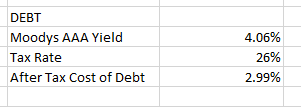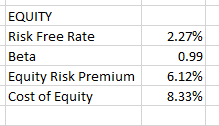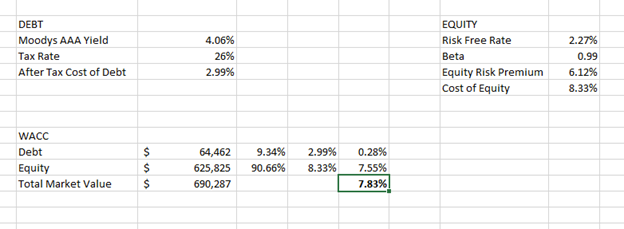1. Products
2. Data Tags & Calculations

# How to Calculate WACC in Excel

## Here is a tutorial to lead you through calculating WACC (weighted average cost of capital) using Intrinio’s Excel add-in.

#### Installation

Begin by installing the Intrinio Excel add-in on your computer. The add-in can be installed on Mac OS X or Microsoft Windows.

#### Setup

Name your sheet in Excel "WACC." Choose a ticker and enter it in cell A1 - so that it can be referenced throughout the entire model. In this article, we’ll be using AAPL as an example.

Click on the A1 Cell, then click on the named range box just above it. Delete "A1" and type the word "ticker." Now, in each of your formulas you'll be able to simply type "ticker" instead of "AAPL" or "A1."

In your WACC worksheet, type the following to get set up:

• "DEBT" into cell D4
• "EQUITY" into cell J4
• "WACC" into cell D11

#### Cost of Debt

Underneath "Debt," type the following into cells D5-D7:

• Moody's AAA Yield
• Tax Rate
• After Tax Cost of Debt

In cell E5, we'll use the Intrinio formula that pulls in Moody's AAA Yield from the Federal Reserve. This percentage represents the riskiness of investment grade corporate bonds. In the case of AAPL we'll use the AAA curve because it represents the highest rated corporates yield to maturity, and AAPL carries a lot of cash and is unlikely to go bankrupt. However, depending on the riskiness of the company you have chosen, you can also pull in the BBB curve.

In cell E5 type the following formula to pull in Moody's AAA Yield:

=IntrinioDataPoint("FRED.AAA","value")/100

We divide by 100 to transform the return value into a percentage.

In cell E6 type the following formula to pull in the Tax Rate:

=IntrinioDataPoint(ticker,"efftaxrate")

To calculate the after-tax cost of debt, we need to take the AAA Yield times (1-tax rate).

In cell E7, to calculate the After-Tax Cost of Debt, type in the following formula:

=E5*(1-E6)

We calculate the cost of debt "after" taxes because interest expense is tax deductive and dividends paid is not. This means there is a tax advantage to taking out debt.

Your Cost of Debt section should look something like this:#### Cost of Equity

Underneath "Equity", type the following into cells J5-J8:

• Risk Free Rate
• Beta
• Cost of Equity

In cell K5, we'll use the Intrinio formula to pull in the risk-free rate from the Federal Reserve:

=IntrinioDataPoint("FRED.DGS10","value")/100

This is the 10-year treasury constant maturity

In cell K6, type the following formula to pull in the Beta for AAPL:

=IntrinioDataPoint(ticker,"beta")

To calculate the Equity Risk Premium, we use data from Aswath Damodaran, a finance professor at the Stern School of Business at New York University. At Intrinio, we call him the Godfather of Value Investing. He is a recognized authoritative source for equity risk premium data.

In cell K7, type in the following formula to pull in Professor Damodaran's ERP value:

=IntrinioDataPoint("DMD.ERP","ttm_erp")

Lastly, to calculate the Cost of Equity, we'll take the Risk Free Rate plus (Beta times the Equity Risk Premium).

In cell K8, type in the following formula to pull in the Cost of Equity:

=K5+(K6*K7)

Your Cost of Equity Section should look something like this:#### WACC

Underneath "WACC", type the following into cells D12-D14:

• Debt
• Equity
• Total Market Value

In cell E12, type the following formula to pull in AAPL's Total Debt:

=IntrinioDataPoint(ticker,"debt")/1000000

In cell E13, type the following formula to pull in AAPL's Total Equity:

=IntrinioDataPoint(ticker,"marketcap")/1000000

In cell E14, type the following formula to calculate AAPL's Total Market Value:

=SUM(E12:E13)

In cell F12, next to the value of debt, we want to calculate debt as a percentage of the total market value.

In cell F12, type the following formula to calculate AAPL's Debt as a percentage of Total Market Value:

=E12/\$E\$14

In cell F13, next to the value of equity, we want to calculate equity as a percentage of the total market value.

In cell F13, type the following formula to calculate AAPL's Equity as a percentage of Total Market Value:

=E13/\$E\$14

In cell G12, next to the debt as a percentage of total market value, type the following formula to pull in the after-tax cost of debt that we previously calculated above:

=E7

In cell G13, next to the equity as a percentage of total market value, type the following formula to pull in the cost of equity that we previously calculated above:

=K8

In cell H12, type in the following formula to calculate the weighted cost of debt:

=F12*G12

In cell H13, type in the following formula to calculate the weighted cost of equity:

=F13*G13

Lastly, in cell H14, type in the following formula to add the two together and calculate the Weighted Average Cost of Capital:

=SUM(H12:H13)

Your WACC worksheet should now look something like this:To learn how to calculate WACC as part of a full DCF valuation, read our DCF valuation case study.